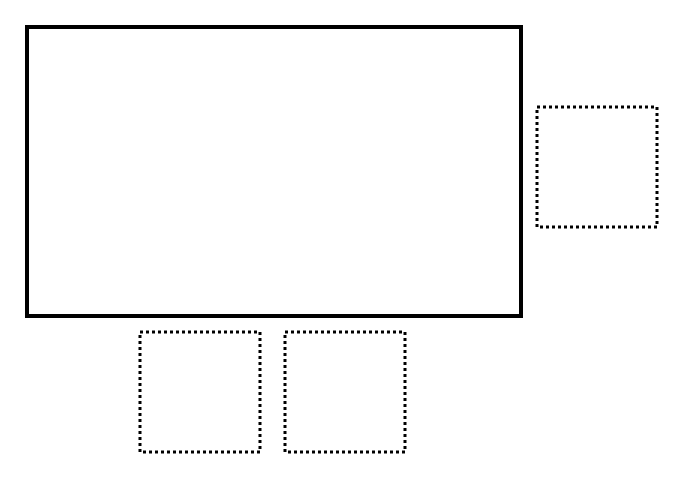# Biggest Rectangle

Directions: Find the largest area for the rectangle filling the boxes with numbers 1 through 9. You may use a digit at most once.### Hint

Which numbers would yield the largest area?

How can you find the area of a rectangle?

Where should you put the largest number?  Why?

What will the rectangle with the largest area look like? (i.e. tall and skinny,…)

### Answer

The area of the largest rectangle is 783 square units with the following dimensions:  9 x 87

Source: Nanette Johnson, Inspired by Mike Chamberlain’s Problem

## Multiply and Divide Within A Hundred 1

Directions: Using the digits 2 to 9 at most one time each, place a digit …